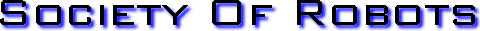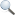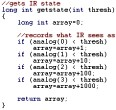Search Here

MISC
Parts List
Robot Forum
Chat
Member Pages
Axon MCU
Robot Books
Shop
Contact

HARDWARE
Actuators
Batteries
Electronics
Materials
Microcontrollers
Sensors

SCIENCE
Robot Journals
Robot Theory
Conferences

PROGRAMMING - VARIABLESC Variables
Controlling variables in your program are very important. Unlike with programming computers (such as in C++ or Java) where you can call floats and long ints left and right, doing so on a microcontroller would cause serious problems. With microcontrollers you need to always be careful about limited memory, limited processing speeds, overflows, signs, and rounding.

C Variable Reference Chart

 definition short int char int unsigned int signed int long int unsigned long int signed long int float bit 2^x 1 -bit 8-bit 8-bit 8-bit 8-bit 16-bit 16-bit 16-bit 32-bit number span allowed 0, 1 (False, True) a-z, A-Z, 0-9 0 .. 255 0 .. 255 -128 .. 127 0 .. 65535 0 .. 65535 -32768 .. 32767 1.2 x 10^(-38) .. 3.4 x 10^(38)

Limited Memory
Obviously the little microcontroller thats the size of a quarter on your robot isn't going to have the practically infinite memory of your PC. Although most microcontrollers today can be programmed without too much worry on memory limits, there are specific instances where it would be important. If your robot does mapping, for example, efficient use of memory is important. You always need to remember to use the variable type that requires the least amount of memory yet still stores the information you need. For example, if a variable is only expected to store a number from 100 to 200, why use a long int when just an int would work? Also, the fewer bits that need to be processed, the faster the processing can occur.

Limited Processing Speeds
Your microcontroller is not a 2.5 GHz processor. Don't treat it like one. Chances are its a 4 MHz to 20 MHz processor. This means that if you write a mathematically complex equation, your microcontroller could take up to seconds to process it. By that time your robot might have collided into a cute squirrel without even knowing!!! With robots you generally want to process your sensor data about 3 to 8 times per second, depending on the speed and environment of your robot. This means you should avoid all use of 16-bit and 32-bit variables at all costs.

You should also avoid all use of exponents and trigonometry - both because they are software implemented and require heavy processing. What if your robot requires a complex equation and there is no way around it? Well, what you would do is take shortcuts. Use lookup tables for often made calculations, such as for trigonometry.

To avoid floats, instead of 13/1.8 use 130/18 by multiplying both numbers by 10 before dividing. Or round your decimal places - speed is almost always more important than accuracy with robots. Be very careful with the order of operations in your equation, as certain orders retain higher accuracy than others. Don't even think about derivatives or integrals.

Overflows
An overflow is when a value for a variable exceeds the allowed number span. For example, an int for a microcontroller cannot exceed 255. If it does, it will loop back.

unsigned int variable = 255;
variable = variable+1; //variable will now equal 0, not 256!!!

To avoid this overflow, you would have to change your variable type to something else, such as a long int. You might also be interested in reading about timers, as accounting for timer overflows is often important when using them.

Signs
Remember that signed variables can be either negative or positive but unsigned variables can only be positive.

In reality you do not always need a negative number. A positive number can often suffice because you can always arbitralily define the symantics of a variable. For example, numbers between 0 and 128 can represent negatives, and numbers between 129 and 255 can represent positive numbers.

But there will often be times when you would perfer to use a negative number for intuitive reasons. For example, when I program a robot, I use negative numbers to represent a motor going in reverse, and a positive for a motor going forward. The main reason I would avoid using negative numbers is simply because a signed int overflows at 128 (or -128) while unsigned overflows at 256 (or 0).

Extras
For further reading of programming variables for robots, have a look at the fuzzy logic tutorial.

Examples of Variables in C Code:

Defining variables:

#define ANGLE_MAX 255//global constants must be defined first in C
int variable = 3;
signed long int constant = -538;

variable math examples (assume answer is reset after each example):

answer = variable / 2; //answer = 1 (because of rounding down)

answer = variable + constant; //answer = 233 (because of overflows and signs)